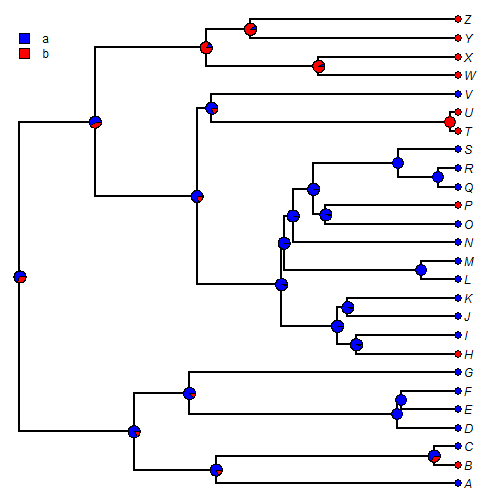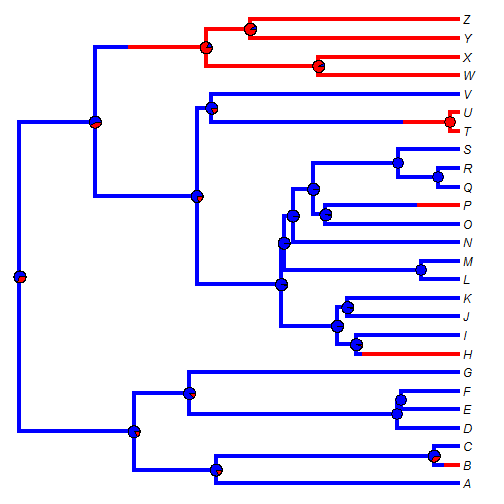## Monday, January 18, 2016

### Reminder on how to overlay posterior probabilities from stochastic mapping on a single 'representative' stochastic map tree

I had a query today about how to overlay the posterior probabilities from stochastic mapping on a single (“representative,” whatever that means) stochastic map tree. An example of this can be seen in Figure 4.3 of Revell (2014): my chapter on plotting methods in a recent edited volume on phylogenetic comparative methods.

It is not too hard. So, having loaded phytools:

``````library(phytools)
``````

imagine we have a phylogeny (`tree`) and a discrete character vector `x`:

``````tree
``````
``````##
## Phylogenetic tree with 26 tips and 25 internal nodes.
##
## Tip labels:
##  A, B, C, D, E, F, ...
##
## Rooted; includes branch lengths.
``````
``````x
``````
``````## A B C D E F G H I J K L M N O P Q R S T U V W X Y Z
## a b a a a a a b a a a a a a a b a a a b b a b b b b
## Levels: a b
``````

Now, let's do stochastic mapping. We have multiple options for this. Here I will use `model="ER"` (equal rates) and `Q="empirical"` (use the MLE of `Q`):

``````mapped.trees<-make.simmap(tree,x,nsim=100,model="ER",Q="empirical")
``````
``````## make.simmap is sampling character histories conditioned on the transition matrix
##
## Q =
##              a            b
## a -0.008508737  0.008508737
## b  0.008508737 -0.008508737
## (estimated using likelihood);
## and (mean) root node prior probabilities
## pi =
##   a   b
## 0.5 0.5
``````
``````## Done.
``````
``````mapped.trees
``````
``````## 100 phylogenetic trees with mapped discrete characters
``````

Now, let's compute the posterior probabilities at all the nodes of the tree. We can do this using the S3 method `summary` for objects of class `"multiSimmap"`:

``````obj<-summary(mapped.trees)
obj
``````
``````## 100 trees with a mapped discrete character with states:
##  a, b
##
## trees have 8.82 changes between states on average
##
## changes are of the following types:
##      a,b  b,a
## x->y 5.9 2.92
##
## mean total time spent in each state is:
##                a           b    total
## raw  709.2151934 336.9705730 1046.186
## prop   0.6779056   0.3220944    1.000
``````

The posterior probabilities at nodes are in `obj\$ace` and we can plot these as follows (once again, using a custom S3 method):

``````colors<-setNames(c("blue","red"),c("a","b"))
plot(obj,colors=colors,ftype="i")
``````Cool. We can also, however, overlay these posterior probabilities on a 'representative' stochastic map tree from the posterior distribution. Let's pick one such tree at random, plot it, and add the posterior probabilities to nodes:

``````i<-sample(1:length(mapped.trees),1)
plot(mapped.trees[[i]],colors=colors,lwd=4,ftype="i")
nodelabels(pie=obj\$ace,piecol=colors[colnames(obj\$ace)],cex=0.6)
``````That's it!